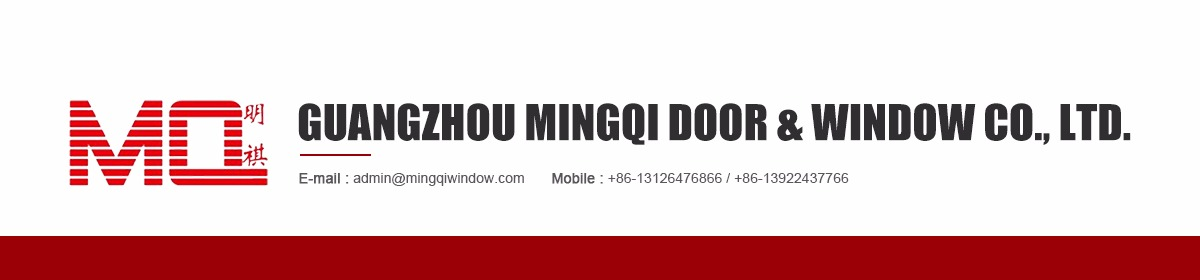• Sourcing Solutions
• Services & Membership
• Help
Orders 0
Favorites 0### Aluminum doors

View as: View list View gallery
Start Order
Place your order online and pay to the designated bank account to get full protection.
Selected Products (0/20)
Click to select products and contact the supplier.
• Min. Order: 1.0 Square Meters
FOB Price: \$35.00 - \$90.00 / Square Meter
• Min. Order: 1.0 Square Meters
FOB Price: \$35.00 - \$90.00 / Square Meter
• Min. Order: 1.0 Square Meters
FOB Price: \$35.00 - \$150.00 / Square Meter
• Min. Order: 5.0 Square Meters
FOB Price: \$50.00 - \$200.00 / Square Meter
• Min. Order: 20 Square Meters
FOB Price: \$50.00 - \$200.00 / Square Meter
• Min. Order: 1 Square Meter
FOB Price: \$50.00 - \$120.00 / Square Meter
• Min. Order: 5 Sets
FOB Price: \$30.00 - \$60.00 / Set
• Min. Order: 5 Sets
FOB Price: \$30.00 - \$60.00 / Set
• Min. Order: 1.0 Square Meters
FOB Price: \$59.99 - \$119.99 / Square Meter
• Min. Order: 1 Square Meter
FOB Price: \$83.00 - \$150.00 / Square Meter
• Min. Order: 5 Square Meters
FOB Price: \$40.00 - \$150.00 / Square Meter
• Min. Order: 10 Square Meters
FOB Price: \$40.00 - \$100.00 / Square Meter
• Min. Order: 5 Square Meters
FOB Price: \$40.00 - \$150.00 / Square Meter
• Min. Order: 10 Square Meters
FOB Price: \$40.00 - \$100.00 / Square Meter
• Min. Order: 1 Square Meter
FOB Price: \$50.00 - \$150.00 / Square Meter
• Min. Order: 5 Square Meters
FOB Price: \$40.00 - \$150.00 / Square Meter
• Min. Order: 5 Square Meters
FOB Price: \$40.00 - \$150.00 / Square Meter
• Min. Order: 1 Square Meter
FOB Price: \$65.00 - \$160.00 / Square Meter
• Min. Order: 5 Square Meters
FOB Price: \$39.00 - \$121.00 / Square Meter
• Min. Order: 1.0 Square Meters
FOB Price: \$35.00 - \$150.00 / Square Meter
• Min. Order: 5 Square Meters
FOB Price: \$40.00 - \$100.00 / Square Meter
• Min. Order: 1.0 Square Meters
FOB Price: \$40.00 - \$90.00 / Square Meter
• Min. Order: 1 Square Meter
FOB Price: \$30.00 - \$160.00 / Square Meter
• Min. Order: 1.0 Square Meters
FOB Price: \$59.99 - \$119.99 / Square Meter
• Min. Order: 1 Square Meter
FOB Price: \$40.00 - \$120.00 / Square Meter
• Min. Order: 1 Square Meter
FOB Price: \$45.00 - \$60.00 / Square Meter
• Min. Order: 1 Square Meter
FOB Price: \$40.00 - \$90.00 / Square Meter
• Min. Order: 1 Square Meter
FOB Price: \$80.00 - \$180.00 / Square Meter
• Min. Order: 1 Square Meter
FOB Price: \$80.00 - \$180.00 / Square Meter
• Min. Order: 1 Square Meter
FOB Price: \$80.00 - \$180.00 / Square Meter
• Min. Order: 1.0 Square Meters
FOB Price: \$45.00 - \$60.00 / Square Meter
• Min. Order: 1 Square Meter
FOB Price: \$45.00 - \$60.00 / Square Meter
• Min. Order: 1 Square Meter
FOB Price: \$45.00 - \$122.00 / Square Meter
• Min. Order: 1 Square Meter
FOB Price: \$80.00 - \$180.00 / Square Meter
• Min. Order: 1 Square Meter
FOB Price: \$40.00 - \$160.00 / Square Meter
• Min. Order: 1 Square Meter
FOB Price: \$45.00 - \$140.00 / Square Meter
• Min. Order: 1 Square Meter
FOB Price: \$30.00 - \$166.00 / Square Meter
• Min. Order: 1 Square Meter
FOB Price: \$45.00 - \$180.00 / Square Meter
• Min. Order: 1.0 Square Meters
FOB Price: \$45.00 - \$60.00 / Square Meter
• Min. Order: 1 Square Meter
FOB Price: \$50.00 - \$100.00 / Square Meter
• Min. Order: 1 Square Meter
FOB Price: \$45.00 - \$180.00 / Square Meter
• Min. Order: 1 Square Meter
FOB Price: \$45.00 - \$180.00 / Square Meter
• Min. Order: 1 Square Meter
FOB Price: \$45.00 - \$180.00 / Square Meter
• Min. Order: 1 Square Meter
FOB Price: \$45.00 - \$180.00 / Square Meter
• Min. Order: 1.0 Square Meters
FOB Price: \$45.00 - \$180.00 / Square Meter
• Min. Order: 1 Square Meter
FOB Price: \$45.00 - \$60.00 / Square Meter
• Min. Order: 1 Square Meter
FOB Price: \$45.00 - \$60.00 / Square Meter
• Min. Order: 1 Square Meter
FOB Price: \$45.00 - \$60.00 / Square Meter
• Min. Order: 5 Square Meters
FOB Price: \$30.00 - \$160.00 / Square Meter
• Min. Order: 5 Sets
FOB Price: \$30.00 - \$50.00 / Set